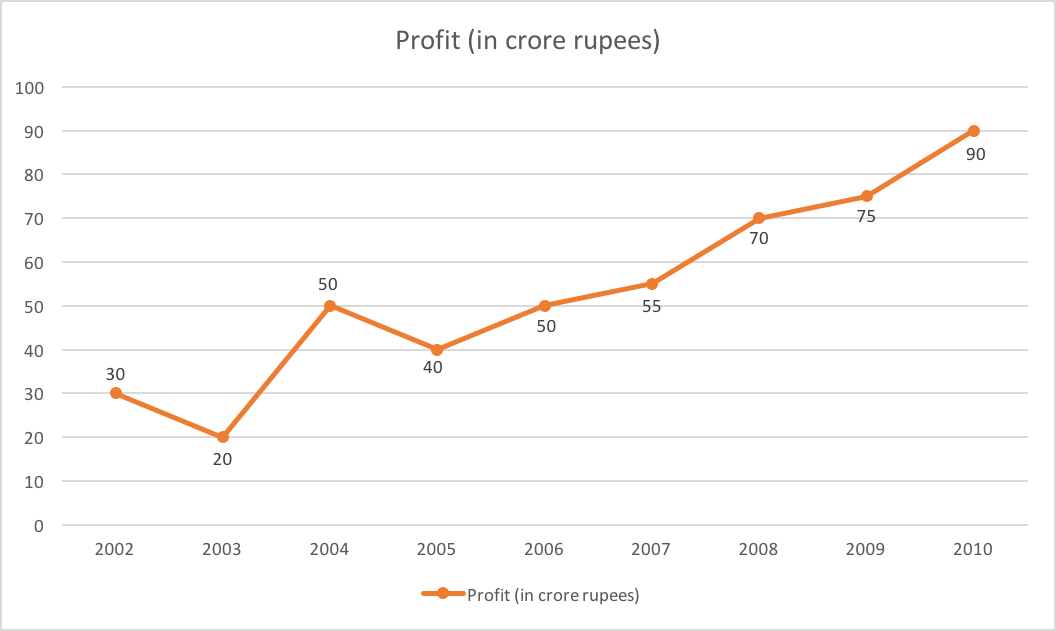## SSC CGL Quant Test 5

Instructions

Study the graph carefully and answer the following questions. Profit Earned by a company (in crore rupees) during certain yearsQuestion 1

If the income in the year 2003 was 60 crores and the expenditure of that year equals the expenditure of the year 2005, the income of the company (in crore rupees) in 2005 was

Question 2

If the expenditure in the year 2007 was 60 crores, then the income (in crore rupees) in that year was

Question 3

The year in which the percentage increase in the profit from that of the previous year is the highest is

Question 4

What is the approximate per cent (to the nearest integer) r,r it of the year 2006, if the inc. —ne of that year was? 120 crores ?

Question 5

What is the approximate per cent (to nearest integer) increase in the profit in the year
2009 from that of the previous year ?